### Paul A. Schwarz, Timothy J. Fahey, and Charles E. McCulloch. 2003. Factors controlling spatial variation of tree species abundance in a forested landscape. Ecology 84:1862–1878.

Appendix A. A description of the statistical analyses used in the study.

Let Z(s) represent the abundance of a species (in this case, ln(basal area + 1)) at a site s defined by the UTM easting and northing coordinates of a field plot. A version of the linear, mixed-effects model without random effects for applications involving a suite of explanatory variables (environmental and disturbance factors) and assuming spatially correlated errors is (in matrix notation)

Z = X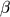+c

where Z is a column vector containing the response variable (i.e., ln(basal area + 1)), X is a matrix of explanatory variables (e.g., environmental factors),is a column vector of unknown, fixed-effects parameters, andc is column vector of normally distributed residuals,c ~ N(0,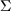). One of the assumptions of the standard least squares regression model is that the residuals have constant variance and are independent and therefore uncorrelated. The linear model with spatially correlated errors is an extension for applications in which the residuals are spatially correlated. It assumes that the residuals are spatially correlated and further, that the spatial correlation can be modeled as a simple function of distance. This spatial correlation structure is described by nonzero off-diagonal elements of the variance-covariance matrix,. These nonzero elements represent the covariance, which can be modeled using a covariogram, C(h), where h is a vector of Euclidean distances between sampling locations and

C(h) = C(0) –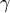(h)

where(h) is the semivariance and C(0) =(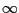) is the variance (the diagonal elements of). The function

2(h) = var[R(s + h) – R(s)]

is the variogram of the residuals, R, where R = ZX. The true form of(h) is usually unknown and therefore must be estimated by fitting one of the accepted semivariogram models to the residuals (for details, refer to Cressie 1993).

For the abundance of each of the tree species in our data (i.e., ln(basal area + 1)), an exponential semivariogram model provided the best fit among the standard models. The exponential model is(h) = c0 + c1[1 – exp(–h/a)],

where c0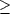0 is the nugget, c10 is the sill, and a0 is the range; h denotes the Euclidean distance between sites.

The semivariogram model parameters (range, sill, and nugget) were estimated using the method of restricted maximum likelihood (REML), which offers several attractive properties for estimating semivariogram parameters. First, parameters estimated via REML are often less biased than those using maximum likelihood, and secondly, REML eliminates the need to subjectively bin the separation distances (lags) into discrete distance classes (Cressie 1993). The NLME library in S-PLUS (Insightful, Inc., Seattle, Washington, USA) offers a convenient means of fitting both the semivariogram models via REML and estimating the fixed effects via generalized least squares; thus, we used S-PLUS and the NLME library to fit each of the spatial linear models (Pinheiro and Bates 2000). The estimated parameters were compared to those produced by the SAS PROC MIXED procedure (SAS 1999), and the parameter estimates were nearly identical.

The significance of individual fixed-effects terms (explanatory factors) in the models was tested using a partial t test as implemented in the NLME library (Pinheiro and Bates 2000). The P values produced by the NLME library were not adjusted for the effects of estimating the spatial autocorrelation structure. However, to address concerns that estimation of the spatial autocorrelation, the moderate sample size, or the non-normality of the abundance data might be inflating the Type I error rate, we performed a Monte Carlo simulation study to determine whether the P values of the significance tests for the explanatory factors in the final models of species abundance required a correction to account for the effects of spatial autocorrelation. In contrast with a partial t test, Monte Carlo simulations do not assume approximate normality, and we expected this approach to provide an accurate, non-asymptotic answer to the question of whether a correction was needed for our data. The study simulated the distribution of the t statistic for each model predictor (explanatory factor) under the null hypothesis that the true value of the predictor was zero. The spatial structure of the actual data was incorporated into the simulated data using the estimated values of the semivariogram parameters for each of the species. In addition, zero values were introduced into the simulated data to better approximate the structure of the actual data. Each of the seven species models was simulated for 1000 iterations. Differences between the P values calculated during the original model fitting process and the P values estimated through Monte Carlo simulation were minimal. The median difference between the original P values and the simulated P values was 0.0003, with 89% of the simulated P values within 0.02 of the original P values (77% were within 0.01). The maximum difference was 0.046 for one of the predictors in the model for B. alleghaniensis, representing a change from P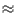0.008 to P0.054. The distribution of small positive and negative differences was balanced, suggesting that the differences were probably a result of noise in the Monte Carlo simulations and not true differences between the original values and the simulations.

The simulation results indicated that the P values from the original model fitting procedure were accurate descriptions of the significance of the individual predictors despite the estimation of the spatial autocorrelation from the abundance data, the moderate sample size, and the non-normality. Most likely, this was because of the relatively large sample size (260 observations) and the modest strength of the spatial autocorrelation present in the residual spatial structure of the abundance data. In no case did the simulation results suggest that one of the predictors should have been dropped from one of the final models. Although the Monte Carlo simulations enabled us to investigate the influence of spatial autocorrelation in our data on the statistical significance of our results, they did not address the general problem of correcting significance tests in multivariable, linear models with spatial correlation; this is the subject of research elsewhere (P. Dutilleul, personal communication).

Literature Cited

Cressie, N. A. C. 1993. Statistics for spatial data (revised edition). John Wiley, New York, New York, USA.

Pinheiro, J. C., and D. M. Bates. 2000. Mixed-effects models in S and S-PLUS. Springer, New York, New York, USA.

SAS. 1999. SAS OnlineDoc, Version 8. SAS Institute, Cary, North Carolina, USA.

[Back to E084-044]